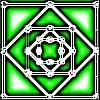You may also likeHomes

There are to be 6 homes built on a new development site. They could be semi-detached, detached or terraced houses. How many different combinations of these can you find?Number Squares

Start with four numbers at the corners of a square and put the total of two corners in the middle of that side. Keep going... Can you estimate what the size of the last four numbers will be?I'm Eight

Find a great variety of ways of asking questions which make 8.

Making Trains

Age 5 to 7 Challenge Level:

Rukmini who goes to Dowanhill Primary sent us a very detailed solution to this problem - well done Rukmini!

I counted how many white rods would fit in Laura's train. Her train was 12 white rods long since it had 7 + 2 + 3 = 12. Yes, I can make trains with 3 different colours, of length 12.
First I took the longest rod Orange (10) but I couldn't do it.
I tried each size one after the other.
I could make the following 8 trains:
Blue + red + white = 9 + 2 + 1 = 12
Brown + white + green = 8+ 1 + 3 = 12
Black + pink + white = 7 + 4 + 1 = 12
Black + green + red = 7 + 3 + 2 = 12
Dark green + yellow + white = 6 + 5 + 1 = 12
Dark green + pink + red = 6 + 4 + 2 = 12
Yellow + dark green + white = 5 + 6 + 1 = 12
Yellow + pink + green = 5 + 4 + 3 = 12

In fact, the fourth one in your list, Rukmini, is the same as Laura's and the fifth and seventh are the same, I think. Do you agree?

After that I did not need to check as the same things would be repeated. That is we would get a train like green + brown + white which is the same as brown + white + green.
The train yellow + dark green + white was 12 long and did not use the same colours as Laura, but was just as long.
Rob's train could have been: 6 + 1 + 2 +3 = 12 : dark green + white + red + green or 5 + 1 + 2 + 4 = 12: yellow + white + red + pink
Charlene's train had the 4 shortest rods: 1 + 2 + 3 +4 = 10. Orange rod is of the same length, 10.
Ben's train was 7 + 7 + 3 = 17 long.
He might have made: pink + yellow + dark green + red = 4 + 5 + 6 + 2 = 17
green + brown + pink + red = 3 + 8 + 4 + 2 = 17
green + black + yellow + red = 3 + 7 + 5 + 2 = 17